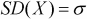# Normal LawThe Normal law is parameterized by a mean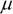(unit: millimeter) and a standard deviation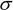(unit: millimeter). Another name for the Normal law is Gaussian law.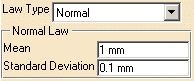Let's take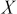a random variable following the Normal law, then:is distributed according to the following density of probability: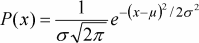With mean: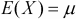With variance: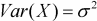With standard deviation: